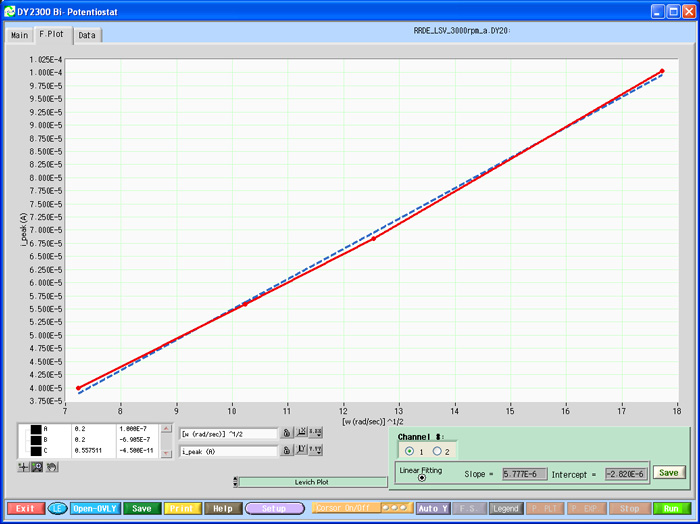## DY2300 Series Bipotentiostat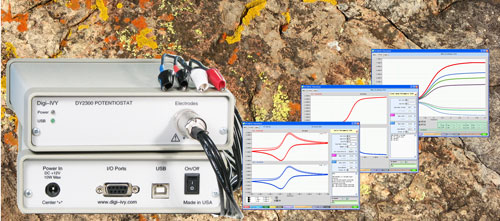## Hardware

 Potential Range ±4.095 V Bias Potential (2nd Channel) ±4.095 V Compliance Voltage ±10.0 V Max. Current Range ±100 mA (±10nA to ±100 mA in 8 steps) Current Resolution 0.002% of the range, 0.3pA for ±10nA range Current Accuracy < 0.5% FSR (1% for 10nA and 100nA range) Input Bias Current @ 25°C < 20 pA Min. Potential Step (user) 1.0 mV Input Impedance of electrometer > 10^12 Ω System Bandwidth > 30 kHz Min. Rise time of Applied Waveform < 5.0 µsec / V Min. Time Base 0.1 msec Max. Data Points 15000 / Channel RRDE Control Voltage Output (V) 0-10 V Digital Output (Stir, Purge) TTL Digital Input (Ext. Trigger) TTL Analog Output (measured Vref & current1) ±10.0 V Cell Connection WE1, WE2, RE, & CE Computer Interface USB with PCs running Windows Dimensions (W x D x H) & Weight 15 x 26 x 5 cm, 1 kg Power Requirements 90-240 VAC, 10 W

## Software Techniques

 Amperometric i-t curve (iT) Sampling Time (sec) = [0.0001 to 100] Cyclic Voltammetry (CV) Scan Rate (V/sec) = [1e-5 to 10] Linear Sweep Voltammetry (LSV) Scan Rate (V/sec) = [1e-5 to 10] Open circuit potential vs. time (OCP) Sampling Time (sec) = [0.0001 to 100] Differential Pulse Voltammetry (DPV) Step E (V) = [0.001 to 0.1], Amplitude (V) = [0.001 to 0.5], Pulse Period (sec) = [0.02 to 100] Normal Pulse Voltammetry (NPV) Step E (V) = [0.001 to 0.5], Pulse Period (sec) = [0.02 to 100] Multi-Step Potential (MSP) Step E (V) = [-2.0, +2.0], Step Width (sec) = [0.005 to 200] Square Wave Voltammetry (SWV) Step E (V) = [0.001 to 0.1], Frequency (Hz) = [0.01 to 50] Chronoamperometry (CA) Pulse Width (sec) = [0.001 to 1000], Sampling Time (sec) = [1e-5, 10] Tafel Plot Anodic (Cathodic) Stripping Voltammetry Run style Single, Auto Repeat, or Auto Sequence

## Sample Plots

•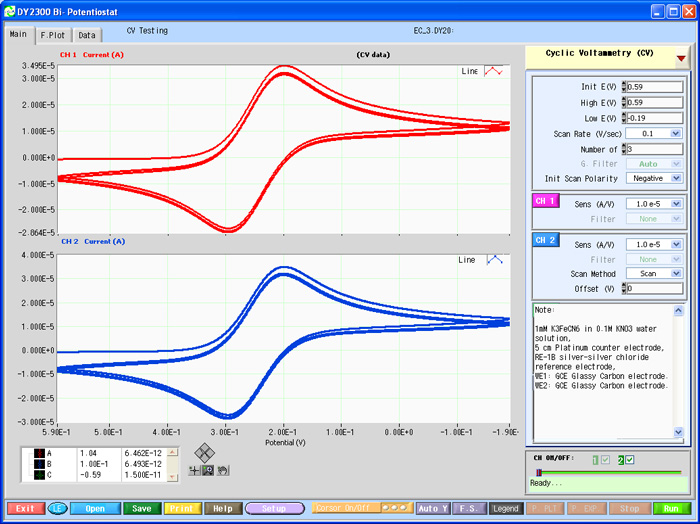•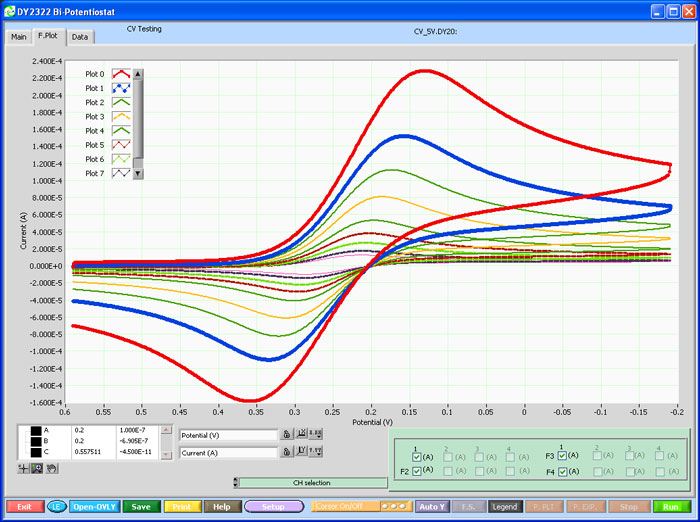•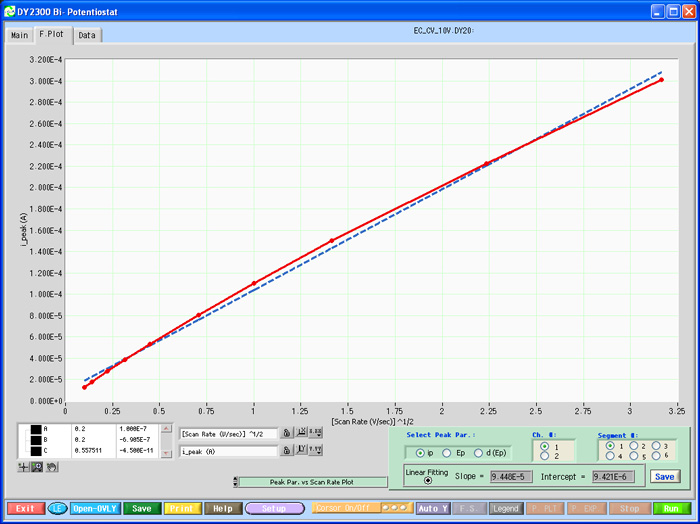••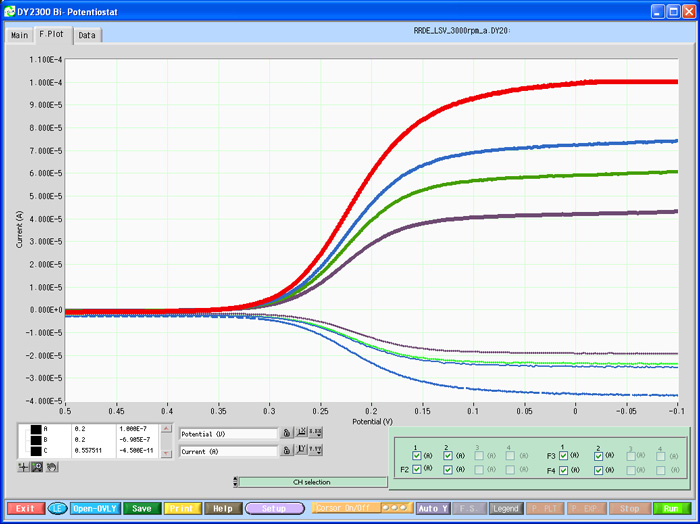•